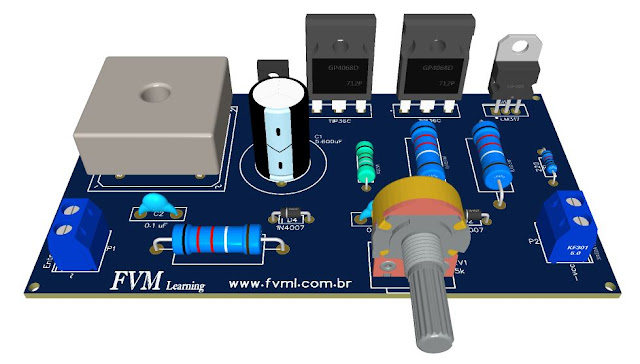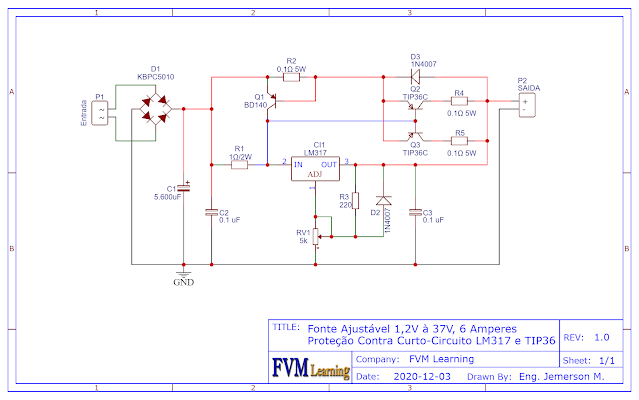## Thursday, October 21, 2021Fig. 1 - Schematic diagram Adjustable power supply circuit with short circuit protection

Para Versão Original em Português, Clique Aqui!

This is an adjustable power supply circuit from 1.2V to 37V and 6 amps of current, with short circuit protection, equipped with adjustable positive voltage stabilization circuits of three terminals LM317, plus a booster circuit, using the TIP36C, which is an inexpensive power transistor.

What makes this power supply special is the implementation of a short-circuit protection circuit, for which a BD140 PNP transistor is used.

## How the circuit works

Resistor R1, which is a load sensing resistor, receives a small current flowing through it. As long as the current in the output circuit does not reach a certain current calculated through R1, the circuit behaves like a normal voltage regulator, because at small "calculated" currents there is no voltage drop in the load sensing resistor, so the Boosters TIP36C transistor does not trip.

As the current in the circuit increases, the voltage across resistor R1 increases. When this voltage reaches about 0.6 V, the "transistor cut-off voltage", the power transistors turn on and current flows through them, with the threshold determined by the maximum current supported by the power transistors.

However, we have implemented a current protection circuit that consists of a circuit equipped with a BD140 transistor with a resistor that acts as a current sensing resistor that serves to polarize the transistor and, depending on the value detected, limit the output current of the entire circuit according to a simple Ohm's Law formula that serves to set this threshold current.

## Formula 1st Ohm's Law

The 1st Ohm's Law states that the potential difference between two points of a resistor is proportional to the electric current flowing in it, and that the ratio of electric potential to electric current is always constant for ohmic resistors. The formula is as follows: V = R * I
• V - Voltage or electric potential
• R - Electrical resistance
• I - Electrical current

Knowing Ohm's Law, we can now calculate the values of the load sense resistors that activate the power stage and the bias resistors of the protection transistors that form the short circuit protection circuit.

First, we need to know the current of the LM317 voltage regulator, which is 1.5 amps according to the datasheet.

LM317 = 1.5A
Let us calculate R1. We know that using Ohm's law, we get the following expression:
• V = R * I
• V = The cut-off voltage of transistors Q2 and Q3 TIP36C is 0.6V. This is the cut-off range of the transistor. Let us call Q2 and Q3 of Qeq

I = This is the current of the regulator IC1. Let us set the operating current of IC1 to 600mA, which is 0.6A. This current is enough for the IC to work unhindered.

### Then:

• R1 = Vbe_Qeq / I_CI1
• R1 = 0.6V / 0.6A
• R1 = 1 Ohm

## Calculation of the protection circuit resistance

Similarly, we need to know the total current of the selected power supply so that there is an interruption in this range. Our power supply for 6 amps.

Power supply = 6A
Let us calculate R2. We know that Ohm's law gives us the following expression:

• V = R * I
• V = The cut-off voltage of the transistor Q1 is 0.6 V. "This is the cut-off range of the transistor".
• I = The total current of the power supply, which is 6A.

### Then:

• R1 = Vbe_Q1 / I_ps
• R1 = 0.6V / 6A
• R1 = 0.1 Ohm

## Current of the power transistors

Q2 + Q3 = 25A + 25A = 50A

However, the total power of the TIP36C transistor is 125W, which means it operates at a current of 25A to 5V. Remember the above formula, P = V * I;
• P = 5V * 25A = 125W.

For this circuit with a maximum voltage of 37V and transistors with a maximum power of 125W, we look as follows:
• Pmax = V * I:
• Imax = P / V = > Imax = 125W / 37V = > Imax = 3.37A
• How are two transistors together Imax = 6,74A

Therefore, our circuit works with two TIP36C transistors to get 6 amps at the output.

Figure 2 shows the schematic of the adjustable power supply circuit with short circuit protection. Those who follow us already know this circuit very well, the difference is exactly in the implementation of the protection circuit, as we can see below.Fig. 2 - Schematic diagram Adjustable power supply circuit with short circuit protection

## Components List

• CI1 ................ Voltage Regulator LM317
• Q1 ................. PNP Transistor BD140
• Q2, Q3 .......... Power Transistor PNP TIP36C
• D1 ................. Bridge Rectifier 50A - KBPC5010
• D2, D3 .......... Rectifier Diode 1N4007
• R1 ................. Resistor 2W / 1Ω
• R2, R4, R5 ... Resistor 5W / 0.1Ω
• R3 ................ Resistor 1/4W / 220Ω
• C1 ................ Electrolytic Capacitor 5600uF - 50V
• C2, C3 .......... Polyester/Ceramic Capacitor 0.1uF or 100nF
• RV1 .............. Potentiometer 5KΩ
• P1, P2 ........... Screw Terminal Type 5mm 2-Pin Connector
• Others .......... Wires, solders, printed circuit board, etc.
Source: fvml.com.br